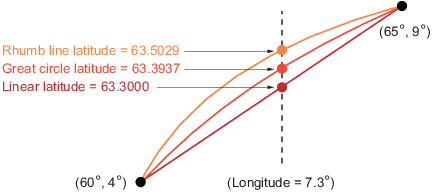## Geographic Interpolation of Vectors

When using vector data, remember that, like raster data, coordinates are sampled measurements. This involves unavoidable assumptions concerning what the geographic reality is between specified data points. The normal assumption when plotting vector data requires that points be connected with straight line segments, which essentially indicates a lack of knowledge about conditions between the measured points. For lines that are by nature continuous, such as most rivers and coastlines, such piecewise linear interpolation can be false and misleading, as the following figure depicts.

Interpolating Sparse Vector DataDespite the possibility of misinterpretation, circumstances do exist in which geographic data interpolation is useful or even necessary. To do this, use the `interpm` function to interpolate between known data points. One value of linearly interpolating points is to fill in lines of constant latitude or longitude (e.g., administrative boundaries) that can curve when projected.

`interpm` returns both the original data and new linearly interpolated points. Sometimes, however, you might want only the interpolated values. The functions `intrplat` and `intrplon` work similarly to the MATLAB® `interp1` function, and give you control over the method used for interpolation. Note that they only interpolate and return one value at a time. Use `intrplat` to interpolate a latitude for a given longitude. Given a monotonic set of longitudes and their matching latitude points, you can interpolate a new latitude for a longitude you specify, interpolating along linear, spline, cubic, rhumb line, or great circle paths. The longitudes must increase or decrease monotonically. If this is not the case, you might be able to use the `intrplon` companion function if the latitude values are monotonic. The following diagram illustrates these three types of interpolation. The `intrplat` function also can perform spline and cubic spline interpolations.

Three Types of Interpolation